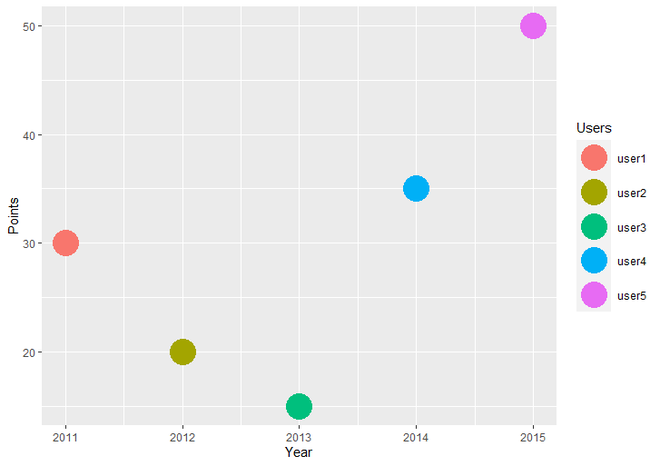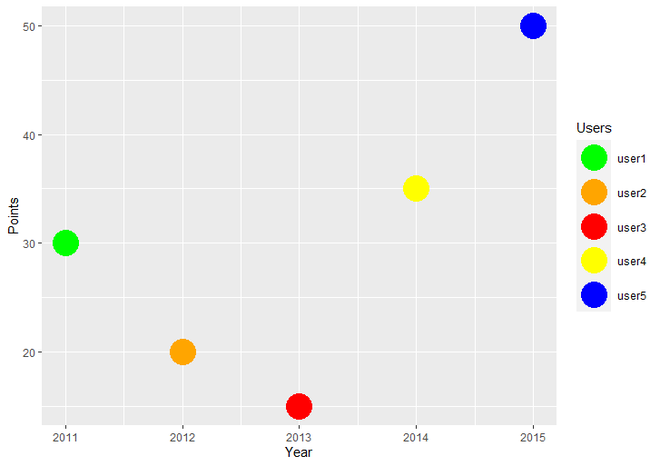# How to Assign Colors to Categorical Variable in ggplot2 Plot in R ?

• Last Updated : 24 Jun, 2021

In this article, we will see how to assign colors to categorical Variables in the ggplot2 plot in R Programming language.

Note: Here we are using a scatter plot, the same can be applied to any other graph.

Dataset in use:

To Create an R plot, we use ggplot() function and to make it scatter plot we add geom_point() function to ggplot() function. By Default, the plot has following colors.

Example:

## R

 `# Load Library``library``(ggplot2)`` ` `# Create DataFrame for Plotting.``data <- ``data.frame``(Year = ``c``(2011, 2012, 2013, 2014, 2015),``                   ``Points = ``c``(30, 20, 15, 35, 50),``                   ``Users = ``c``(``"user1"``, ``"user2"``, ``"user3"``,``                             ``"user4"``, ``"user5"``))`` ` `# Create ggplot2 ScatterPlot.``ggplot``(data, ``aes``(Year, Points, color = Users)) +   ``  ``geom_point``(size = 10)`

Output:ScatterPlot using ggplot2 with default colors

In R Programming, we have many in built function for creating our own discrete scales such as scale_fill_manual, scale_size_manual, scale_shape_manual, scale_linetype_manual, etc. To assign desired colors to categorical data, we use one of them scale_color_manual() function, which is used to scale (map) the manual colors.

Syntax : scale_color_manual(values)

Parameter :

• values : A set of aesthetic values to map the data. Here we take desired set of colors.

Return : Scale the manual values of colors on data.

Example:

## R

 `# Load Library``library``(ggplot2)`` ` `# Create DataFrame for Plotting``data <- ``data.frame``(Year = ``c``(2011, 2012, 2013, 2014, 2015),``                   ``Points = ``c``(30, 20, 15, 35, 50),``                   ``Users = ``c``(``"user1"``, ``"user2"``, ``"user3"``,``                             ``"user4"``, ``"user5"``))`` ` `# Create a ScatterPlot with fixed colors of ``# points(data).``ggplot``(data, ``aes``(Year, Points, color = Users)) +   ``  ``geom_point``(size = 10)+``  ``scale_color_manual``(values = ``c``(``"green"``, ``"orange"``, ``"red"``,``                                ``"yellow"``, ``"blue"``))`

Output :Plot with fixed colors

My Personal Notes arrow_drop_up